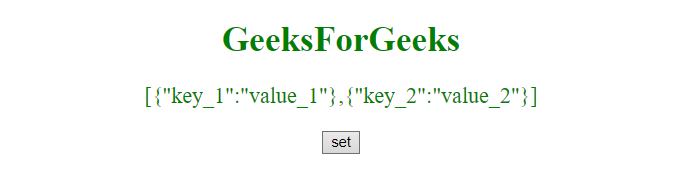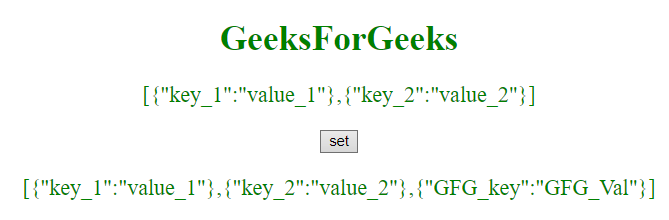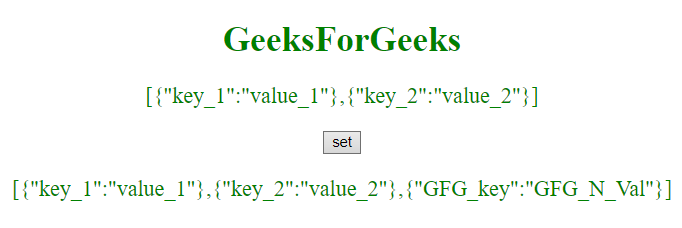# JavaScript | Set object key by variable

In order to set an object’s key by variable, here are few steps to follow.
Steps:

• First make a variable.
• Then make a object.
• Assign that variable a value.

Here are few of the examples.
Example-1: This example sets the object key by variable key and then inserts {“GFG_key”:”GFG_Val”} object to a array.

 ` ` `<``html``> ` ` `  `<``head``> ` `    ``<``title``> ` `        ``JavaScript | Set object key by variable ` `    `` ` ` `  ` ` ` `  `<``body` `style``=``"text-align:center;"``> ` ` `  `    ``<``h1` `style``=``"color:green;"``>   ` `            ``GeeksForGeeks   ` `        `` ` `    ``<``p` `id``=``"GFG_UP"` `       ``style="color:green; ` `              ``font-size: 20px;"> ` `    `` ` ` `  `    ``<``button` `id``=``"GFG_Button"`  `            ``onclick``=``"set()"``> ` `      ``set ` `  `` ` `    ``<``p` `id``=``"GFG_P"`  `       ``style="color:green; ` `              ``font-size: 20px;"> ` `    `` ` `    ``<``script``> ` `        ``myArray = [{ ` `            ``'key_1': 'value_1' ` `        ``}, { ` `            ``'key_2': 'value_2' ` `        ``}]; ` `        ``var up =  ` `            ``document.getElementById("GFG_UP"); ` `       `  `        ``up.innerHTML =  ` `          ``JSON.stringify(myArray); ` `       `  `        ``var down =  ` `            ``document.getElementById("GFG_P"); ` ` `  `        ``function set() { ` ` `  `            ``var key = "GFG_key"; ` `            ``var obj = {}; ` `            ``obj[key] = "GFG_Val"; ` `            ``myArray.push(obj); ` `            ``down.innerHTML = JSON.stringify(myArray); ` `        ``} ` `    `` ` ` ` ` `  ` `

Output:

• Before clicking on the button:• After clicking on the button:Example-2:This example sets the object key by variable key as well as object value by variable val and then inserts {“GFG_key”:”GFG_N_Val”} object to a array.

 ` ` `<``html``> ` ` `  `<``head``> ` `    ``<``title``> ` `        ``JavaScript | Set object key by variable ` `    `` ` ` `  ` ` ` `  `<``body` `style``=``"text-align:center;"``> ` ` `  `    ``<``h1` `style``=``"color:green;"``>   ` `            ``GeeksForGeeks   ` `        `` ` `    ``<``p` `id``=``"GFG_UP"` `       ``style="color:green;  ` `              ``font-size: 20px;"> ` `    `` ` ` `  `    ``<``button` `id``=``"GFG_Button"` `            ``onclick``=``"set()"``> ` `      ``set ` `  `` ` `    ``<``p` `id``=``"GFG_P"` `       ``style="color:green; ` `              ``font-size: 20px;"> ` `    `` ` `    ``<``script``> ` `        ``myArray = [{ ` `            ``'key_1': 'value_1' ` `        ``}, { ` `            ``'key_2': 'value_2' ` `        ``}]; ` `        ``var up = document.getElementById("GFG_UP"); ` `        ``up.innerHTML = JSON.stringify(myArray); ` `        ``var down = document.getElementById("GFG_P"); ` ` `  `        ``function set() { ` ` `  `            ``var key = "GFG_key"; ` `            ``var obj = {}; ` `            ``var val = "GFG_N_Val"; ` `            ``obj[key] = val; ` `            ``myArray.push(obj); ` `            ``down.innerHTML = JSON.stringify(myArray); ` `        ``} ` `    `` ` ` ` ` `  ` `

Output:

• Before clicking on the button:• After clicking on the button:My Personal Notes arrow_drop_upCheck out this Author's contributed articles.

If you like GeeksforGeeks and would like to contribute, you can also write an article using contribute.geeksforgeeks.org or mail your article to contribute@geeksforgeeks.org. See your article appearing on the GeeksforGeeks main page and help other Geeks.

Please Improve this article if you find anything incorrect by clicking on the "Improve Article" button below.